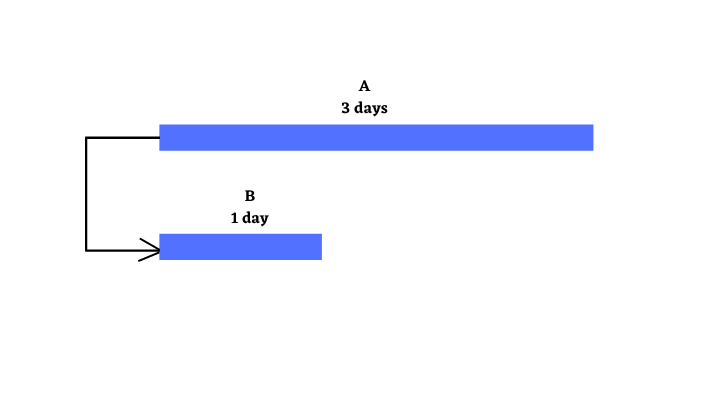Start To Start is a logical relationship (or dependency) in which a successor activity cannot start until its predecessor activity has started.

Start to Start (SS) is one of the four activity relationships of project management. These are used while preparing project schedule. The other three relationships are:

1. FS Relationship
2. FF Relationship
3. SF Relationship

Let us understand SS relationship in greater depth by using examples, Gantt Chart, Network Diagram, and Mathematical formulas.

## Start to Start Relationship (Dependency) in Project Management

As per the PMBOK Guide “Start to Start is a Logical Relationship in which a Successor Activity cannot start until a Predecessor Activity has started”.

However a better definition is, “Start to Start is a Logical Relationship in which starting event of a Successor Activity is dependent on the starting event of a Predecessor Activity”.

To understand the above definition, let us look at the events of an activity and not the whole activity. The two distinct events of any activity are:

1. Start Event (S)
2. Finish Event (F)

In SS relationship, the Start event of the Second Activity is dependent on the Start event of First Activity. The Second Activity is called the Successor and the First Activity is called the Predecessor.

You can also look at Max Wideman’s Glossary for a complete set of PDM definitions.

Note: An activity or task relationship is loosely termed as task dependency. However, these two terms are different. A relationship between two activities can be established only if one activity is dependent on the other. Just like relationships, there are four type of task dependencies.

## Start To Start Examples

Let us consider two activities X and Y. X and Y are predecessor and successor activities respectively. The following examples show SS relationship between X and Y:

• X – Write Code for S/W Module Alpha, Y – Write DB Scripts for S/W Module Alpha
• X – Pour Asphalt, Y – Level Road
• X – Write User Manual, Y – Review User Manual

## Representation of Start To Start

### Gantt Chart

An SS relationship can be visually depicted using using Time Scaled Bar Charts, which are popularly known as Gantt Charts.

Let us consider two activities A and B.

• Duration of A is 3 days.
• Duration of B is 1 day.
• B has a SS relationship with A

The Gantt Chart below shows SS relationship between A and B.As per the above diagram B’s Start is dependent on A’s Start, which means that B can Start(S) as soon as A Starts(S).

In the above example, A and B together are scheduled to be completed in 3 days.

### Project Network Diagram

An SS relationship can also be drawn by using Precedence Diagramming Method (PDM), which can be visually depicted by Network Diagrams.

Let us again consider the above two activities A and B. The Network Diagram below shows SS relationship between A and B.### Mathematical Formulas

Again considering the above two activities A and B, we can mathematically represent the relationship between A and B as:

B(S) = A(S)

The formula will remain same whether we use 0 method or 1 method for solving network diagrams

SS relationships can be further modified by introducing Lead and Lag scheduling modifiers.

The following Lead and Lag examples use 0 method representation.

Two activities J and K having SS relationship with 1 day of Lead:

K(S) = J(S) – 1 day

Two activities L and M having SS relationship with 2 days of Lag:

M(S) = L(S) + 2 days

### Over to You

PDM Relationships can be represented by using mathematical equations. However, not all mathematical relationships represent the natural logic. While making a project schedule, you should ensure that all dependencies follow the natural logic and are true in the real world.

Do you think SS relationship is useful in real projects? Have you ever used it in you project(s)? Or have you seen it being used?

I would love to hear from you.

### PMP Exam Formulas

I have also compiled a PMP Formulas Cheat Sheet. It contains 45 formulas and 57 abbrviations. It will help you in your exam prep. It is the best and most comprehensive cheat sheet based on the PMBOK Guide 6th edition. You can download it free of cost for your studies.

If you are looking beyond a cheat sheet, then I would suggest you to buy detailed PMP Exam Formula Study Guide by Cornelius Fichtner. It contains detailed explanations of all the formulas along with examples and 105 practice questions.

Disclosure: This article contains affiliate links - it means that, if you buy from any of these links, then I will receive a small commission that would help me in maintaining this blog for free. However, for you, there is no extra cost. I recommend only those products that I believe will definitely help the certification aspirants.

1. Anna says:

great!